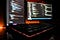# Introduction to the Big O NotationPhoto by Fotis Fotopoulos on Unsplash

“In computer science, big O notation is used to classify algorithms according to how their run time or space requirements grow as the input size grows.” — Wikipedia.

In simple words, Big O notation theoretically explains how fast a piece of code is and how much memory it is using. Before we dive deep into Big O, we need to understand a couple of terms: time complexity and space complexity.

Time Complexity: Time complexity is the number of operations an algorithm/code requires to complete its task. The fewer operations it takes, the faster the algorithm is. By defining the N number of operations performed on an algorithm, Big O Notation expresses its run time regarding how quickly it grows relative to the input ’n’. As the input n grows, so grows the number of operation N. If this definition sounds intimidating, don’t worry; we will explore some examples.

One question that came to my mind when I first heard about time complexity is why we don’t use execution time to compare which algorithm is faster. It makes sense to track the execution time to compare algorithms, right? Well, there are few issues if we use runtime.

• Execution time may vary from machine to machine. Powerful hardware will run the same algorithm faster than a less powerful machine.

That’s why Big O uses input size and the number of times a code is executed to measure the time complexity.

Space Complexity: Space complexity measures the total amount of memory an algorithm/code requires to run. Sometimes you will hear the term “Auxiliary space complexity,” which refers to the space needed for the algorithm, excluding the space taken up by the inputs. For our Big O discussion, we are mainly concerned with the auxiliary space complexity.

Now that we understand the terms time and space complexities let’s understand the different time and space complexities.

Different time complexities

A nifty trick to remember when determining the complexities is we are concerned about the big picture. What will happen when the input size grows huge? If there are few constant operations, we will ignore that. For example, if an algorithm has (4 + n) or (5 * n) number of operations where n is the input size, we will focus on n and remove the constant 4 and 5 out of sight!

1. Constant time O(1)

A constant time or O (1) is when the runtime does not change with the input size.

2. Linear time O(n)

An algorithm is said to have linear time complexity of O (n) when the number of operations grows linearly with the input length. Linear means if input increases by one, then operations also increase by one.

3. Quadratic time O(n²)

If the input size quadratically increases the number of operations, it has an O (n ^ 2) time complexity. For example, if the input size is 2, it will do 4 operations. If the input size is 4, then it will do 16 operations. Usually, if you have a nested for loops, it will run in O (n²) complexity.

For the above example, if we run the quadratic function with the array [1,2,3], then the inner loop will run 3 times (length of the array) for every array element. So, for 3 elements, it will run 3*3 = 9 times.

4. Logarithmic time O (log n)

When an algorithm reduces the input’s size in each step, it is said to have a logarithmic time complexity. In this case, the number of operations is not equal to the size of the input. As the size of the input becomes larger, the number of operations decreases.

Different Space Complexities

1. Most primitives (Booleans, numbers, undefined, null) are constant space.

Here, num has a constant or O(1) space complexity, but the arr has O(n) space complexity because as the input array grows, the arr will grow accordingly.

Before I wrap up, here are few takeaways:

1. When we measure the performance of an algorithm, we are concerned about the worst case. What would happen when the input grows really big?

Full stack developer experienced in Ruby and JavaScript based programming.

## More from Muazzam Nashat

Full stack developer experienced in Ruby and JavaScript based programming.